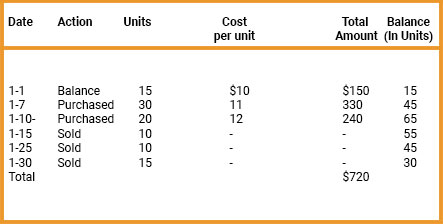The commonly used inventory methods are FIFO (First-In, First-Out), LIFO (Last-In, First-Out) and Weighted Average. When calculating inventory and cost of goods sold, there are two truths that will always hold. The total goods available for sale is equal to the sum of the ending inventory and the cost of goods sold. Using the data in the diagram, that means that ending inventory, plus the cost of goods sold will be equal to \$720. Likewise the sum of the units in ending inventory and the units in cost of goods sold will total 65. By checking these numbers each time ending inventory and cost of goods sold is calculated, minor arithmetic errors are quickly identified.

### FIFO

The First-in, first-out inventory method assumes that costs are charged against revenue in the order in which they were incurred. Thus the most recent costs are assumed to still be in inventory.As can be seen in the diagram, total units is equal to 65 (30 in ending inventory and 35 in cost of goods sold). Also the sum of the ending inventory and cost of goods sold is equal to \$350 + \$370 = \$720.

### LIFO

The Last-In, First-Out inventory method assumes that costs are charged against revenue in the reverse order in which they were incurred. Thus the oldest costs are assumed to still be in the inventory.As can be seen from the example, total units is equal to 65 (30 in ending inventory and 35 in cost of goods sold). Also the sum of the ending inventory and cost of goods sold is equal to \$315 + \$405 = \$720.

LIFO is allowed for income tax reporting purposes. However, if LIFO is used for income tax purposes, it must also be used for financial reporting purposes.

### Weighted Average

The weighted average method is also sometimes called the average cost method. This method assumes that the same cost per unit is charged to units remaining in inventory as was charged to units that have been sold during the period. The calculations for this method are much simpler than those for FIFO and LIFO.

Using the same data, we find that the total cost of \$720 divided by the total units of 65 gives a unit cost of \$11.0769. Then the calculations for ending inventory and cost of goods sold are:

Ending inventory = 30 units x \$11.0769 = \$332.31

Cost of goods sold = 35 units x \$11.0769 = \$387.69

Therefore, the total units is equal to 65 (30 in ending inventory and 35 in cost of goods sold). In addition, the sum of the ending inventory and cost of goods sold is equal to \$332.31 + \$387.69 = \$720.

SingaporeAccounting.com provide bookkeepingaccounting for startups, small business, SMEs and MNCs. We can be reached at  68174500 or enquiry@singaporeaccounting.com.

Call Now Button